# MomentJS - Start of Time

Start of Time method will set the display to the start of the unit given.

## Syntax

```moment().startOf(String);
```

## Example using year as the input

```var day = moment().startOf('year');
```

This will display the first day of the year and will set the time, that is hours, minutes, seconds to 00:00:00 as shown below −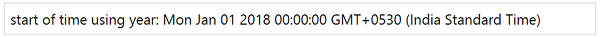## Example using month as the input

```var day = moment().startOf('month');
```

This will display the first day of the month and will set the time, that is hours, minutes and seconds to 00:00:00 as shown below −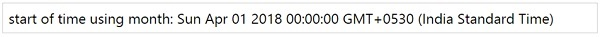## Example using quarter as the input

```var day = moment().startOf('quarter');
```

This will give the details of the start of the current quarter. Time will be set to 12 am.

## Output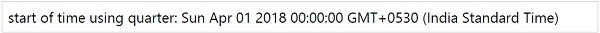If we check the quarter for the month of February using the command shown here, the output will be from 1st of Jan as shown below −

```var day = moment().startOf('quarter');
```

## Output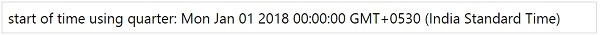## Example using week as the input

```var day = moment().startOf('week');
```

## Output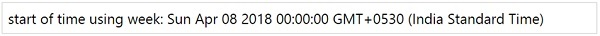momentjs_manipulate_date_and_time.htm